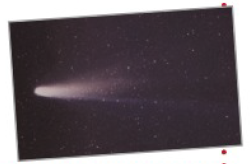Chapter 10.1, Problem 77E

Chapter
Section
Textbook Problem

# Halley’s CometProbably the most famous of all comets, Halley’s comet, has an elliptical orbit with the sun at one focus. Its maximum distance from the sun is approximately 35.29 AU (1 astronomical unit is approximately 92.956 × 10 6 miles), and its minimum distance is approximately 0.59 AU. Find the eccentricity of the orbit.To determine

To calculate: The eccentricity of elliptical orbit of Halley’s Comet, where the nearest and farthest distance of the Sun from the Halley’s Comet is 0.59 AU and 35 AU respectively.

Explanation

Given:

The nearest and farthest distance of the Sun from Halley’s Comet is 0.59 AU and 35 AU respectively.

Formula used:

The eccentricity of ellipse, e=ca.

Calculation:

Consider an elliptical orbit for Halley’s Comet such that, 2a is the length of major axis and 2b is the length of minor axis.

Let farthest and closest point on an elliptical curve from foci of an ellipse are A and P respectively. Let c is radius of Halley’s Comet.

So, the nearest point from Halley’s Comet is,

A=ac …...…... (1)

The farthest point from the Halley’s Comet is,

P=a+c …...…... (2)

Add equation (1) and equation (2),

A+P=2aa=A+P2

Put the value of a in equation (2), to find the value of c,

P=a+cc=aP=A+P2P

### Still sussing out bartleby?

Check out a sample textbook solution.

See a sample solution

#### The Solution to Your Study Problems

Bartleby provides explanations to thousands of textbook problems written by our experts, many with advanced degrees!

Get Started